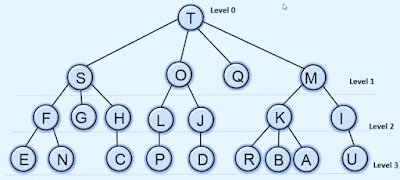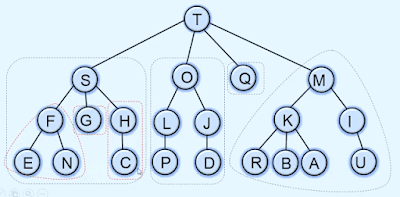A tree is a nonlinear data structure that represents the hierarchical relationship among its elements.

## What is a tree in Data structure?

A tree is a finite set of nodes such that there is always a distinguished node called a root. and other nodes are partitioned into subtrees.### Types of trees in data structures

1. Binary tree
2. Strictly binary tree
3. Extended binary tree
4. Full binary tree
5. Complete binary tree

but before we get into details of each one of these trees first we need to understand some basic terms about trees.

#### What is Node

Each element of the tree is called a node of the tree. as you see in the above image every element like S, O, and Q is a node.

#### What is Edge

The lines connecting the nodes are called edges. like the line connecting the node T and S is called an edge of the tree.

#### What is Parent Node

The immediate predecessor of a node is called the parent node. like T is the parent of nodes S, O, Q and M. because T is the immediate predecessor of nodes S, O, Q and M.

#### What is a child node?

The immediate successor of a node is called the child node. like the nodes S, O, Q, and M are child nodes of Node T.

#### What is the root node?

A node that doesn't have any parent node is called the root node. like Node T doesn't have any parent node so it is a root node of the tree.

#### What is the Leaf node?

The node that does not have any child is called a leaf node. like the nodes, E and N don't have any child nodes so both are called leaf nodes.

#### What is the level of the tree?

The distance of that node from the root is called the level of the node. here in the example, there are four levels.#### What is the Height of the tree?

The total number of levels in a tree is called the height of the tree. like in the above example tree has four levels so the height of the tree is four.

#### What are Siblings Nodes?

Two or more nodes that have the same parent node are called sibling nodes. like the nodes, E and N have the same parent node F so both are siblings of each other.

#### What is the Path in the tree?

The sequence of nodes in which each node is the parent of the next node is called the path in the tree. like in the above example path TSFE is a path in the tree.

#### What is the Ancestor node?

Node A is the ancestor of node B if node A lies in the unique path from the root node to node B. like in the above example node S is the ancestor node for F, G, H, E, N, and C nodes.

#### What is the Descendent node?

If node A is the ancestor of node B then B is the Descendent node of node A. like in the above example nodes F, G, H, E, N and C are descendent nodes for node S.

#### What is Subtree?

A subtree is a part of the tree and in a tree, there is a minimum of one subtree.#### What is the Degree of the node?

The number of subtrees or children of a node is called the degree of a node. so in the above example node tree has a degree of 3 because the maximum number of children is 3 that is connected to node T.